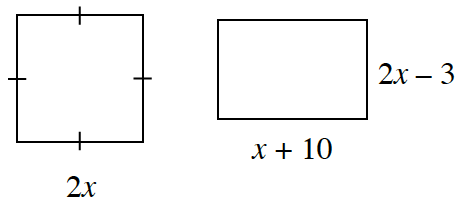### Home > CCG > Chapter Ch8 > Lesson 8.2.1 > Problem8-76

8-76.

If the rectangles below have the same area, find x. Is there more than one answer? Show all work.If the areas are the same, how can you set up an equation to solve for $x$?

Based on this equation, can there be two answers?
Remember with the area of a rectangle being base $\times$ height, you end up with $(2x)(2x) = (x + 10)(2x − 3)$.